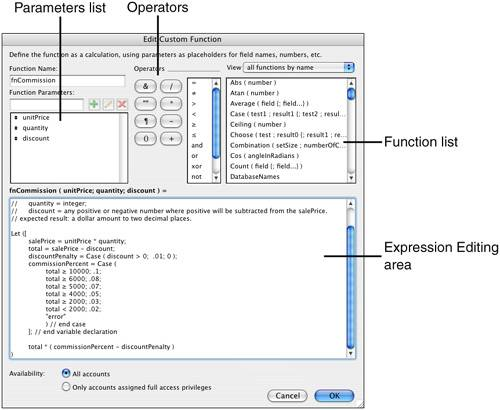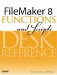# The Custom Function Interface

##### Figure 7.1. The Custom Function dialog allows developers to define parameters that then serve as input for an expression that is then written to reference those parameters.#### Custom Functions: Things to Remember

Custom functions work much like calculations, but its important to understand the following aspects of custom functions:

• Custom functions follow the same rules for syntax that calculation functions follow.For a review of calculation syntax, see Chapter 4 "Working with Calculations Primer."

• Instead of referencing schema information (data fields), custom functions make use of parameters. Values for these parameters are passed into the custom function from elsewhere in ones database via the calculations dialog.
• Custom functions return a single result.
• Custom functions cannot directly incorporate use of container data.
• Writing custom functions requires FileMaker Pro Advanced; however, once written, anyone with access to a calculation dialog can make use of a custom function.
• Custom functions can make use of all the functions built into FileMaker, including other custom functions and external functions.
• It is possible to make use of schema data fields by using the Evaluate() function. The following example illustrates a scenario where a sales commission is referenced:

``` fnSalesCommission ( unitPrice; quantity; discount )
// function to calculate the sales commission for various transaction totals.
// expected input:
// unitPrice = dollar amount to two decimal places;
// quantity = integer;
// discount = any number (positive = discount)
// expected result: a dollar amount.

Let ([
salePrice = unitPrice * quantity;
total = salePrice - discount;
discountPenalty = Case ( discount > 0; .01; 0 );
commissionPercent = Evaluate ( "ProductRate::Commission" ) - discountPenalty
];// end variable declaration
total * ( commissionPercent - discountPenalty )
)
```
• Just as custom functions can reference other functions, they can reference themselves as well. This allows you to write recursive functions in FileMaker.

If no exit condition exists or an error in logic occurs, the maximum number of recursions a function can make is 50,000 deep. If you create multiple branches of recursion, the most branches a custom function can perform is 10,000.

The following is an example of a simple recursive function that reorders a carriage returndelimited list from bottom to top:

``` fnListBackwards ( valueList )
// function to reverse-order a ¶-delimited list
// expected input:
// valuelist = text values delimited by ¶
// expected result: a valuelist of text values delimited by ¶ in reverse order

Let ([
numOfValues = PatternCount ( valuelist; "¶" );
firstValue = LeftValues ( valuelist; 1 )
remainingList = RightValues ( valuelist; numOfValues - 1 );
resultList = Case ( numOfValues = 1; ""; fnListBackwards ( remainingList ) );
];// end variables
resultList & firstValue

)
```
• Custom functions can interact with global and local variables. In the case of local variables (\$myVar), FileMaker will store values specific to a currently running script. In the case of a global variable (\$\$myVar), the value in the variable will be updated and maintained throughout the file.

If you wish to create or set variables within a custom function, use the Let() function:

``` Let ([
myInternalVariable = \$\$globalVariable + 1;
\$\$newVariable = 1 + 1;
result = \$\$newVariable + myInternalVariable
]; // end variables
result
)
```

Custom functions are extremely powerful tools for building abstract units of logic that can then be reused throughout a solution. Indeed, once a custom function has been created (and tested!) its easy enough to recreate them in other files and use them throughout all your solutions. We strongly recommend you create a library of tools to refine and reuse over time. To that end, visit the next chapter for a selection of some of our favorites.FileMaker 8 Functions and Scripts Desk Reference
ISBN: 0789735113
EAN: 2147483647
Year: 2004
Pages: 352